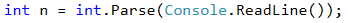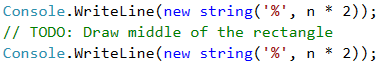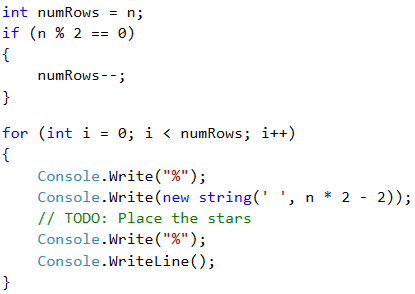Problem: Rectangle with Stars in the Center

The next sample exam problem is about using nested loops and calculations to print on the console a rectangle of given size with stars in the middle, like the ones shown below in the examples.

Video: Rectangle with Stars in the Center

Watch the video lesson about solving the "Rectangle with Stars in the Center" problem: https://youtu.be/6cOJDJm6sNk.

Problem Description

Write a program that reads from the console an integer n and draws a rectangle with size n with two asterisks is its center as in the examples below.

Input

The input is an integer n in the range [2 … 1000].

Output

The rectangle should be printed on the console as in the examples below.

Sample Input and Output

Input Output Input Output
2 %%%%
%**%
%%%%
3 %%%%%%
%    %
% ** %
%    %
%%%%%%
Input Output Input Output
4 %%%%%%%%
%      %
%  **  %
%      %
%%%%%%%%
5 %%%%%%%%%%
%        %
%        %
%   **   %
%        %
%        %
%%%%%%%%%%Printing the First and the Last Rows

The first thing we can easily notice is that the first and last rows contain 2 * n symbols %. We will start with this and then draw the middle part of the rectangle.Printing the Middle Rows

From the examples we see that the middle part of the figure always has odd number of rows. Note that when an even number is set, the number of rows is equal to the previous odd number (2 -> 1, 4 -> 3, etc.). We create a variable that represents the number of rows that our rectangle will have, and correct it if the number n is even. Then we will draw a rectangle without the asterisks. Each row has for the beginning and the end the symbol % and between them 2 * n - 2 empty spaces (the width is 2 * n and we subtract 2 for the two percent at the end). Do not forget to move the code for the last line after the loop.We can start and test the code so far. Everything without the two asterisks in the middle should work correctly.

Adding Stars in the Center of the Rectangle

Now, in the body of the loop let's add the asterisks. We'll check if we're on the middle row. If we are in the middle, we will draw the row together with the asterisks, if not – we will draw a normal row. The line with the asterisks has n-2 empty spaces (n is half the length and we remove the asterisk and the percentage), two stars and again n-2 empty spaces. We leave out of the check the two percent at the beginning and at the end of the row.